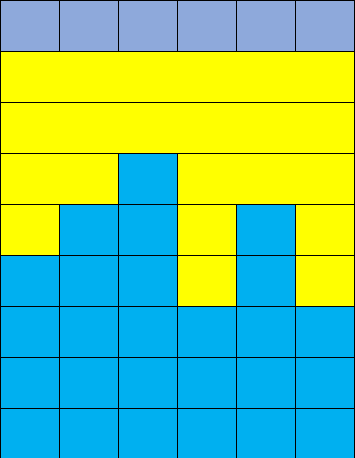# NOIP2018 提高组题解

$$1\leq n\leq 1e5,0\leq d_i\leq 1e4$$

### Thoughts & Solution（图中最上面一行为地面，黄色部分每一块就是一次填充）很显然这样就是最优方案。

//Author: RingweEH
int n,las,ans;

int main()
{
for ( int i=1,x; i<=n; i++ )
{
if ( x>las ) ans+=(x-las);
las=x;
}

printf( "%d\n",ans );

return 0;
}


## Day1-T2 货币系统(money)

$$n$$ 种不同面值的货币，第 $$i$$ 种面值为 $$a[i]$$ ，每种无限张。

$$n\leq 100,a[i]\leq 25000$$ .

### Thoughts & Solution

Proof

Q.E.D.

• 如果枚举到 $$a[i]$$ 时，$$f[a[i]]=1$$ ，那么直接计入答案并跳过即可；
• 如果没有，那么枚举所有的 $$j=a[i]\sim mx$$$$f[j]|=f[j-a[i]]$$ （就是用 $$j-a[i]$$$$a[i]$$ 组成 $$j$$ ，枚举范围中的 $$mx$$ 表示所有 $$a[i]$$ 中的最大值）

//Author: RingweEH
const int N=110,M=25010;
int n,a[N],f[M];

int main()
{
while ( T-- )
{
for ( int i=1; i<=n; i++ )

sort( a+1,a+1+n ); int mx=a[n];
memset( f,0,sizeof(f) ); f=1; int ans=n;
for ( int i=1; i<=n; i++ )
{
if ( f[a[i]] ) { ans--; continue; }
for ( int j=a[i]; j<=mx; j++ )
f[j]|=f[j-a[i]];
}

printf( "%d\n",ans );
}
}


## Day1-T3 赛道修建(track)

$$2\leq n\leq 5e4,1\leq m\leq n-1$$ .

### Thoughts & Solution

//Author: RingweEH
const int N=5e4+10;
struct edge
{
int to,nxt,val;
}e[N<<1];
vector<int> son[N];

void add( int u,int v,int w )
{
}

int get_pair( int u,int pos,int num,int lim )       //在去掉pos这个数之后，所能匹配出的最大对数
{
int now_res=0,l=0;
for ( int r=num-1; r; r-- )
{
r-=(r==pos);
while ( l<r && son[u][l]+son[u][r]<lim ) l++;
l+=(l==pos);
if ( l>=r ) break;
now_res++; l++;
}
return now_res;
}

void dfs( int u,int fa,int lim )
{
son[u].clear();
for ( int i=head[u]; i; i=e[i].nxt )
{
int v=e[i].to; if ( v==fa ) continue;
dfs( v,u,lim );  f[v]+=e[i].val;
if ( f[v]>=lim ) res++;
else son[u].push_back( f[v] );
}
//将所有子树上来的链拉到根节点，并判断是否可以独自产生贡献，如果不能那么放入配对序列
int num=son[u].size(); sort( son[u].begin(),son[u].end() );
int l=0,r=num-1,cnt=0;
for ( ; r; r-- )
{
while ( l<r && son[u][l]+son[u][r]<lim ) l++;
if ( l>=r ) break;
cnt++; l++;
}
//配对过程，cnt就是得到的最大对数。
res+=cnt;
if ( cnt*2==num ) { f[u]=0; return; }       //全配完了，不需要再往上转移
l=0; r=num-1; int now_res=0;
while ( l<=r )
{
int mid=(l+r)>>1,now=get_pair( u,mid,num,lim );
if ( now==cnt ) now_res=mid,l=mid+1;
else r=mid-1;
}
//二分转移上去的最大链长，使得剩下的链仍能满足最大匹配
f[u]=son[u][now_res];
}

bool check( int mid )
{
memset( f,0,sizeof(f) );
res=0; dfs( 1,0,mid );
return (res>=m) ? 1 : 0;
}

int main()
{
freopen( "track.in","r",stdin );  freopen( "track.out","w",stdout );

for ( int i=1,u,v,w; i<n; i++ )

int l=0,ans=0; r=r/m;
while ( l<=r )
{
int mid=(l+r)>>1;
if ( check(mid) ) ans=mid,l=mid+1;
else r=mid-1;
}

printf( "%d\n",ans );

fclose( stdin ); fclose( stdout );
return 0;
}


## Day2-T1 旅行(travel)

$$n\leq 5e3$$ , $$m=n$$$$m=n-1$$ .

### Thoughts & Solution

#### 40pts 基环树

C++ 之父：STL常数大那是你编译器不行（

//Author: RingweEH
const int N=5010;
int n,m,pos=0,cnt=0,better;
bool vis[N];
vector<int> g[N],path,ans;
pair<int,int> edge[N];

void dfs( int u )
{
if ( (!better) && (u>ans[cnt]) ) return;
if ( !better && u<ans[cnt] ) better=1;
vis[u]=1; path.push_back( u ); cnt++;
for ( int i=0; i<g[u].size(); i++ )
{
int v=g[u][i];
if ( vis[v] ) continue;
if ( (u==edge[pos].first) && (v==edge[pos].second ) ) continue;
if ( (v==edge[pos].first) && (u==edge[pos].second ) ) continue;
dfs( v );
}
}

int main()
{
freopen( "travel.in","r",stdin ); freopen( "travel.out","w",stdout );

edge.first=edge.second=-1;
for ( int i=1,u,v; i<=m; i++ )
{
edge[i].first=u; edge[i].second=v;
}

for ( int i=1; i<=n; i++ )
sort( g[i].begin(),g[i].end() );
for ( int i=1; i<=n; i++ )
ans.push_back( 60000 );
if ( m==n )
{
for ( int i=1; i<=m; i++ )
{
memset( vis,0,sizeof(vis) ); path.clear();
pos=i; cnt=better=0; dfs( 1 );
if ( cnt==n ) ans=path;
}
}
else
{
dfs( 1 );
if ( cnt==n ) ans=path;
}

for ( int i=0; i<ans.size(); i++ )
printf( "%d ",ans[i] );

fclose( stdin ); fclose( stdout );
return 0;
}


## Day2-T2 填数游戏(game)

• 用一个格子的行列坐标来表示格子，坐标从 0 开始编号
• 合法路径起点是 $$(0,0)$$ 终点是 $$(n-1,m-1)$$ ，每次只能向下移动一格或者向上移动一格，向下走是 D 向上走是 R .
• 对于一条合法路径 $$P$$ ，用字符串 $$w(P)$$ 表示其走向，用 $$s(P)$$ 表示路径经过的所有数组成的序列
• 合法的填数方案满足：对于两条合法路径 $$P_1,P_2$$ ，如果 $$w(P_1)>w(P_2)$$ ，那么 $$s(P_1)\leq s(P_2)$$ .（大小按字典序排）

## Day2-T3 保卫王国(defense)

• 由道路直接连接的两座城市中至少要有一座城市驻扎军队。
• 在编号为 $$i$$ 的城市中驻扎军队的花费是 $$p_i$$

### Thoughts & Solution

$$f[u]$$$$u$$ 不放军队的子树最小代价，$$f[u]$$ 类似。

$f[u]=\sum_{v\in son[u]}f[v]\\\\ f[u]=a[u]+\sum_{v\in son[u]}\min(f[v],f[v])$

$f[u]=g[u]+f[wson[u]]\\\\ f[u]=g[u]+\min(f[wson[u]],f[wson[u]])$

$\begin{bmatrix} \infin & g[u]\\ g[u] & g[u] \end{bmatrix} \begin{bmatrix} f[wson]\\ f[wson] \end{bmatrix} = \begin{bmatrix} f[u]\\ f[u] \end{bmatrix}$

//Author: RingweEH
//LOJ#2955. 「NOIP2018」保卫王国
#define ls tr[x]
#define rs tr[x]
const int N=1e5+10;
const ll INF=2e15;
ll val[N];
map<int,int> mp[N];
struct Matrix
{
ll mat;
ll get_mx() { return max(mat,mat); }
Matrix( ll x=-INF,ll y=-INF ) { mat=mat=x; mat=y; mat=-INF; }
void Set( ll x=-INF,ll y=-INF ) { mat=mat=x; mat=y; mat=-INF; }
}g[N];
Matrix operator * ( Matrix A,Matrix B ) {}
struct Edge { int to,nxt; }e[N<<1];
bool IsRoot( int x ) { return (tr[fa[x]]==x) || (tr[fa[x]]==x); }
void Pushup( int x )
{
g[x].Set(val[x],val[x]);
if ( ls ) g[x]=g[ls]*g[x];
if ( rs ) g[x]=g[x]*g[rs];
}
void Rotate( int x )
{
int y=fa[x],z=fa[y]; bool kid=(tr[y]==x);
if ( IsRoot(y) ) tr[z][tr[z]==y]=x;
tr[y][kid]=tr[x][kid^1]; fa[tr[x][kid^1]]=y; tr[x][kid^1]=y; fa[y]=x; fa[x]=z;
Pushup(y); Pushup(x);
}
void Splay( int x )
{
int y,z;
while ( IsRoot(x) )
{
y=fa[x]; z=fa[y];
if ( IsRoot(y) )
Rotate( ((tr[y]==x)^(tr[z]==y)) ? x : y );
Rotate(x);
}
}
void Access( int x )
{
for ( int y=0; x; y=x,x=fa[x] )
{
Splay(x);
if ( rs ) val[x]+=g[rs].get_mx(),val[x]+=g[rs].mat;
if ( y ) val[x]-=g[y].get_mx(),val[x]-=g[y].mat;
tr[x]=y; Pushup(x);
}
}

void DFS( int u,int fat )
{
fa[u]=fat; val[u]=w[u];
for ( int i=head[u]; i; i=e[i].nxt )
{
int v=e[i].to;
if ( v==fat ) continue;
DFS(v,u); val[u]+=val[v]; val[u]+=max(val[v],val[v]);
}
g[u].Set(val[u],val[u]);
}

int main()
{
scanf( "%d%d",&n,&m ); char typ; scanf( "%s",typ ); ll sum=0;
for ( int i=1; i<=n; i++ ) w[i]=read(),sum+=w[i];
for ( int i=1,u,v; i<n; i++ )

DFS(1,0);
for ( int i=1; i<=m; i++ )
{